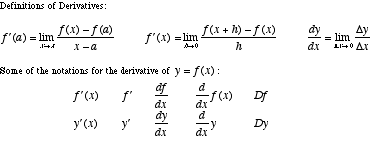index: click on a letter A B C D E F G H I J K L M N O P Q R S T U V W X Y Z A to Z index index: subject areas numbers & symbols sets, logic, proofs geometry algebra trigonometry advanced algebra & pre-calculus calculus advanced topics probability & statistics real world applications multimedia entrieswww.mathwords.com about mathwords website feedback

 Derivative A function which gives the slope of a curve; that is, the slope of the line tangent to a function. The derivative of a function f at a point x is commonly written f '(x). For example, if f(x) = x3 then f '(x) = 3x2. The slope of the tangent line when x = 5 is f '(x) = 3·52 = 75.See also